# Convergence, almost-certain

almost-sure convergence, convergence with probability one

Convergence of a sequence of random variables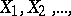defined on a certain probability space, to a random variable, defined in the following way: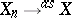(or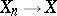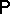-almost certain) if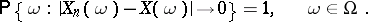In mathematical analysis this form of convergence is called almost-everywhere convergence. Convergence in probability follows from almost-certain convergence.